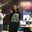Trusted answers to developer questions
Trusted Answers to Developer Questions

Related Tags

# What is the conjunctive normal form?Ayesha Kanwal

Grokking Modern System Design Interview for Engineers & Managers

Ace your System Design Interview and take your career to the next level. Learn to handle the design of applications like Netflix, Quora, Facebook, Uber, and many more in a 45-min interview. Learn the RESHADED framework for architecting web-scale applications by determining requirements, constraints, and assumptions before diving into a step-by-step design process.

### Overview

The conjunctive normal form is a way of expressing a formula in the boolean logicA branch of algebra in which the values of the variables are either true or false only.. It is also called the clausal normal form.

### Conjunctive normal form

The conjunctive normal form states that a formula is in CNF if it is a conjunction of one or more than one clause, where each clause is a disjunction of literals. In other words, it is a product of sums where $\wedge$ symbol occurs between the clauses and the $\vee$ symbol occurs in the clauses.

### Steps to convert a formula into CNF

• We eliminate all the occurrences of $⊕$ (XOR operator), $\rightarrow$ (conditional), and $↔$ (biconditional) from the formula. We convert it into its equivalent formula containing $\vee$, $\wedge$ , and $\neg$ symbol. We use the following logical equivalences:
• $A ⊕ B \equiv (A \vee B) \wedge \neg (A \wedge B)$
• $A \rightarrow B \equiv \neg A \vee B$
• $A ↔ B \equiv (\neg A \vee B) \wedge (A \vee \neg B)$
• $A ↔ B \equiv (A \wedge B) \vee (\neg A \wedge \neg B)$
• We move all the negations inwards to appear only as a part of the literal. The $\neg$ symbol can only precede a propositional variable or a predicate symbol. To accomplish this, we use the following logical equivalences:
• $\neg \neg A \equiv A$
• De Morgan's law
• Some common equivalences that we use for the conversion are:
• Commutativity for disjunction: $A \vee B \equiv B \vee A$
• Commutativity for conjunction: $A \wedge B \equiv B \wedge A$
• Associativity for disjunction: $(A \vee B) \vee C \equiv A \vee (B \vee C)$
• Associativity for conjunction: $(A \wedge B) \wedge C \equiv A \wedge (B \wedge C)$
• Distribution over disjunction: $A \vee (B \wedge C) \equiv (A \vee B) \wedge (A \vee C)$
• Distribution over conjunction: $A \wedge (B \vee C) \equiv (A \wedge B) \vee (A \wedge C)$

### Example

Consider the first formula,

Remove the conditional symbol as per the rules explained in the first step:

Remove the double negation as mentioned in the second step:

Apply the De Morgans law that opens a negation as a whole:

Remove the double negation similar to the step we did before:

Apply the De Morgans law again:

Remove the double negation again:

Apply the distributive property over disjunction, which will lead to two clauses:

This is the final conjunction form of the formula. This is in the product of sums form.

Let's take another formula:

Remove all the occurrences of the conditional symbols:

Apply the distributive property over conjunction:

Apply the same property:

By the rules of disjunction, $\neg B \vee B$ is true:

Using the identity property for conjunction, $A \wedge T$ is $A$:

Finally, using the distributive property over conjunction:

Hence, the final conjunctive form.

Note: Both the final forms have a conjunctive symbol between the clauses, while a disjunctive symbol is used in the clauses.

RELATED TAGS

CONTRIBUTORAyesha Kanwal